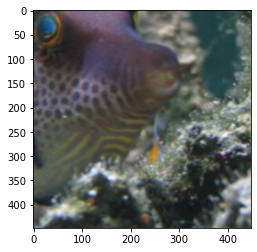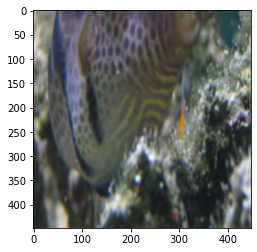# The affine transformation parameters obtained by cv2.getaffinetransform are not correct

I am training a network similar to Spatial Transformer Networks, which is used to estimate affine transformation parameters according to the incoming heatmap. The labels are the parameters of affine transformation through the coordinates of the target box. So I tried cv2.getaffinetransform to get the parameters of the corresponding affine transformation, but it seemed that the final result was not ideal.

# Code:

This code is to display the bbox and (minx,miny,maxx,maxy) is the coordinates of the bbox

``````miny=127
minx=191
maxx=351
maxy=351
print(miny,minx,maxx,maxy)
fig, ax = plt.subplots(ncols=1, nrows=1, figsize=(6, 6))
ax.imshow(image)
rect=patches.Rectangle((minx, miny), maxx - minx, maxy - miny,
fill=False, edgecolor='red', linewidth=2)
``````And I get the parameters :

``````points1=np.float32([[191,127],[191,351],[351,351]])
points2=np.float32([[0,0],[0,448],[448,448]])
M=cv2.getAffineTransform(points2,points1)
M

``````

Finally, I use the steps of affine transformation in Spatial Transformer Networks to transform：

``````theta=torch.from_numpy(M).float()
grid=F.affine_grid(theta.unsqueeze(0),tensor_image.shape)
grid=grid.cuda()
output=F.grid_sample(tensor_image,grid)

``````You can see that the affine transformation is not exactly the region of the target box

Is there something wrong with my code？

I guess there might be a mismatch between the x and y coordinates in the image coordinate system and the rows and columns in `affine_grid`. Could you try to swap the coordinates of the axes and rerun the code?

I’m sorry to reply you so late. I tried to exchange the axis, but the final result was not very good either

``````points1=np.float32([[127,191],[351,191],[351,351]])
points2=np.float32([[0,0],[448,0],[448,448]])
M=cv2.getAffineTransform(points2,points1)
M

``````I wonder if it’s a matter of paneling, because the paneling in M that cv2 gets is the pixel value, and the paneling in affine_grid is supposed to be proportional, so I deal with paneling in this way

The image shape is 448*448

``````M=M/448
M=M/448
``````